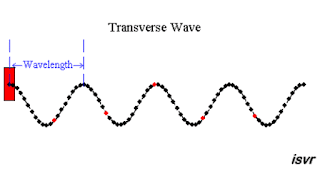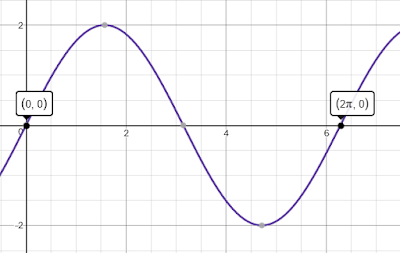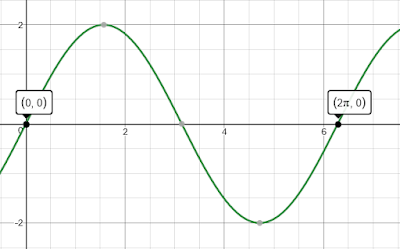## Pages

### 4.2 Deriving the speed of wave.

In this notes we'll give a short derivation of the speed of the wave.

Let's consider the following wave motion,Image Courtesy: isvr.soton.ac.uk/span>
As you know that as time changes, the waves propagates. Propagation of wave for one complete cycle is the point from $(0,0)$ to the point $(2\pi,0)$, as shown below. Remember that the plot below is the snap shot(of the animated wave propagation above) at two instances when the point was at $(0,0)$ and the other instances when it reached to the point $(2\pi,0)$. The distance covered during this propagation is a one wavelength i.e. $(\lambda)$,Position-Displacement plots

We also know that this propagation is due to the oscillation of the water molecule (as shown in the red dot in the above animation) at any given point. During this propagation the water molecule completes a one complete oscillation (up and down), that is time taken for one complete wave propagation to take place and is equal to the time taken for one complete oscillation of the water molecules $(T)$. The plot of the displacement-time for oscillation is given below,Displacement-Time plot
In simple term distance $\lambda$ (one wave length) takes T amount of time.

So since
\begin{align*}
v&=\frac{distance}{time}=\frac{\lambda}{T} \\&=\lambda f \end{align*}

Thus, the speed of wave propagation is given by,
$$v=\lambda f$$
Here's a demonstration video,

$Ex.$ Given a sound wave of frequency $400Hz$ and wave length of $0.8m$. Calculate the speed of the propagating sound wave.(ans:$320ms^{-1}$)